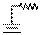### Create an Account

Home / Questions / The circuit of Figure P826 will remove the DC portion of the input voltage v1t while ampli...

# The circuit of Figure P826 will remove the DC portion of the input voltage v1t while amplifying the AC portion Let

The circuit of Figure P8.26 will remove the DC portion of the input voltage v1(twhile amplifying

the AC portion. Let v1(t) = 10 + 10−3 sin ωV,

RF  = 10 k2, and Vbatt  = 20 V.

a.    Find Rsuch that no DC voltage appears at the output.

b.    What is vout(t), using Rfrom part a?RFRS

Jun 18 2020 View more View LessSubscribe To Get Solution